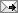BIG NEWS

## Saturday, December 04, 2010

### Ordering Pizza

Pizza Hutt has three sizes of pizza: regular, large and big. David Farrar asks, which costs the most -the big pizza or the large one? I'd ask which one is the best value for money.

It transpires that the large pizza is not bigger than the big pizza. That's because the large pizza, at \$12.90 has eight slices. The big pizza is actually 33.3 percent bigger than the large pizza as it has 12 slices, (assuming all pizza slices are the same size). That makes the big pizza twice as big as the six slice regular pizza, but at \$15.90, it is much less than twice the price of the regular pizza, so it is better value for money. The large pizza is better value than the big pizza as well , as it is less than 33.3 percent more expensive then the big pizza. At \$1.32 a slice it is the best value for money.

This means that while three (smaller) large pizzas have the same number of pieces as two ( bigger) big pizzas, at \$38.70 that is \$7.20 more than the two big pizzas cost. If you want a better deal, go to Dominos, where eight slice pizzas are a lot less than \$10.90. On a good night you can get five large pizzas - usually called regular at Dominos - for a few bucks more than two big Pizza Hut pizzas.

Aternatively, you can go to Hell. Where an equivalent pizza will cost more than one at Dominos, but the biggest one costs less than the big one at Pizza Hutt, but more than the large one.And the large one is the same size as the regular Dominos one which costs even less. Wonder if the size of the slices are the same in all three stores?

Labels:Scoopit!